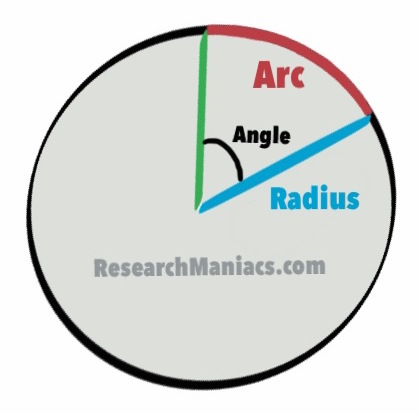Here we will show you how to convert 90 Degrees to radians. 90 Degrees is a measurement of an angle. Radians is the ratio of the arc length to the radius of a circle. See Figure 1.

On this page, we put 90 Degrees into our formula to calculate the radians. The formula for converting degrees to radians is as follows:

Radians = (π / 180) x Degrees

If we put 90 Degrees into our formula we get: (π / 180) x 90 =

1.5707963267949

Extra credit: Many people also want to know what 90 Degrees is in radians expressed in terms of Pi. The math would be (90/180)π and the answer is as follows: 0.5000π

Figure 1This page shows how to convert an 90 degree angle to radians, where radians is the ratio of the arc length to the radius of the circle. Although not the correct dimensions, the figure above shows the parts of the circle and triangle we are working with.